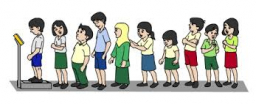# (arithmetic 59613

Calculate the average number of children per woman (arithmetic mean of the number of children) in a certain workgroup of women. The following numbers of children were found: 0, 1, 3, 2, 2, 1, 0, 3, 1, 1, 0, 1.

m =  1.25

### Step-by-step explanation:Did you find an error or inaccuracy? Feel free to write us. Thank you!

Tips for related online calculators
Looking for help with calculating arithmetic mean?
Looking for a statistical calculator?

#### Grade of the word problem:

We encourage you to watch this tutorial video on this math problem: